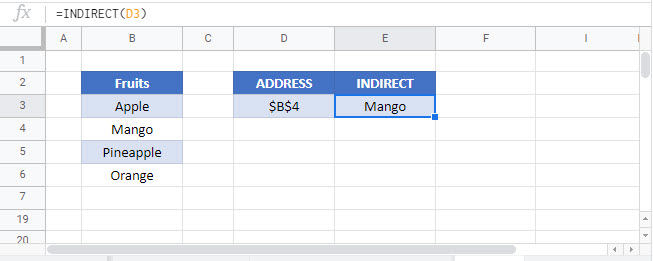# Get Cell Value by Address (Row & Column) – Excel & Google Sheets

This tutorial demonstrates how to get a cell value using the address of the cell (row and column) in Excel and Google Sheets.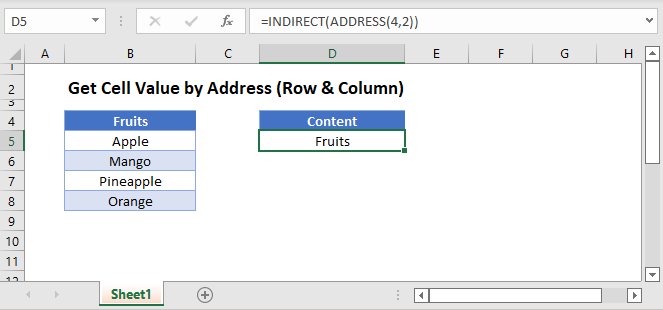## Get Cell Value With the INDEX Function

We can get the value of a cell (its content) by using the INDEX Function.

The INDEX Function looks up a cell contained within a specified range and returns its value.

``=INDEX(A1:J10,C3,C4)``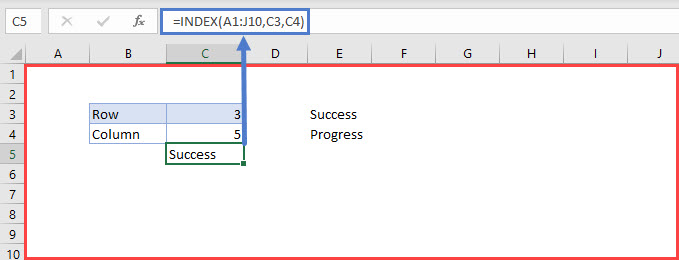In the example above, the range specified in the INDEX formula is “A1:J10”, the row is cell “C3” (“3”), and the column is cell “C4” (“5”).

The formula looks through the range “A1:J10”, checks the cell in Row 3 and Column 5 (“E3”) and returns its value (“Success”).

## Get Cell Value With the INDIRECT Function

Another option for getting the value of a cell by its address is to use the INDIRECT Function.

We can use the INDIRECT Function here in two different ways.

### Using Text Reference Within the INDIRECT Function

The INDIRECT Function changes a specified text string to a cell reference and returns its value.

``=INDIRECT("R" & 3 & "C" & 2,FALSE)``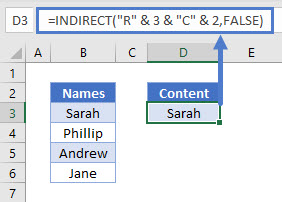In the example above, the specified text string is “R3C2”. This means Row 3, Column 2. So the formula returns the value in that address (“Sarah”).

Note: The FALSE argument in this formula indicates the cell reference format (R1C1). (TRUE would use A1 style cell referencing).

### Get Cell Value by Using INDIRECT and ADDRESS

The second way to get the value of a cell using the INDIRECT Function is to combine it with the ADDRESS Function.

``=INDIRECT(ADDRESS(4,2))``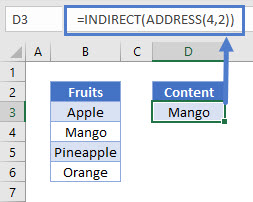Let’s breakdown the formula into steps.

``=ADDRESS(4,2)``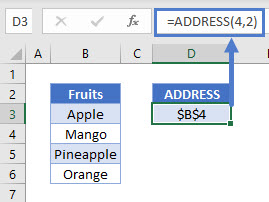The ADDRESS Function takes a specified row number (“4”) and column number (“2”) and returns its absolute reference (“\$B\$4”). Therefore, the absolute reference for the cell in Column 2 (Column B based on position) and Row 4 is \$B\$4.

The INDIRECT Function returns the value of the cell referenced.

``=INDIRECT(D3)``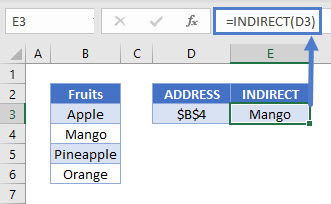Combining these steps gives us our initial formula:

``=INDIRECT(ADDRESS(4,2))``Note: The INDIRECT Function is volatile. It recalculates every time the workbook does and can cause Excel to run slowly. We recommend avoiding the use of large numbers of INDIRECT Functions in your workbooks.